International
Tables for
Crystallography
Volume B
Reciprocal space
Edited by U. Shmueli

International Tables for Crystallography (2010). Vol. B, ch. 1.5, pp. 178-179   | 1 | 2 |

## Section 1.5.3.4. Irreducible representations of space groups and the reciprocal-space group

M. I. Aroyoa* and H. Wondratschekb

aDepartamento de Fisíca de la Materia Condensada, Facultad de Cienca y Technología, Universidad del País Vasco, Apartado 644, 48080 Bilbao, Spain , and bInstitut für Kristallographie, Universität, D-76128 Karlsruhe, Germany
Correspondence e-mail:  wmpararm@lg.ehu.es

#### 1.5.3.4. Irreducible representations of space groups and the reciprocal-space group

| top | pdf |

Letbe a conventional basis of the lattice L of the space group. From (1.5.3.6),and, equation (1.5.3.4)can be writtenEquation (1.5.3.12)has the same form if a primitive basisof L has been chosen. In this case, the vector k is given bywith rational coefficients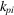.

Let a primitive basisbe chosen for the lattice L. The set of all vectors k (known as wavevectors) forms a discontinuous array. Consider two wavevectors k and, where K is a vector of the reciprocal lattice. Obviously, k anddescribe the same irrep of. Therefore, to determine all irreps ofit is necessary to consider only the wavevectors of a small region of the reciprocal space, where the translation of this region by all vectors offills the reciprocal space without gap or overlap. Such a region is called a fundamental region of. (The nomenclature in literature is not quite uniform. We follow here widely adopted definitions.)

The fundamental region ofis not uniquely determined. Two types of fundamental regions are of interest in this chapter:

 (1) The first Brillouin zone is that range of k space around o for whichholds for any vector(Wigner–Seitz cell or domain of influence in k space). Visually, it is the region of endpoints of the k vectors that are nearer to the origin than to any other point of the reciprocal lattice. The Brillouin zone is a centrosymmetric body bounded by centrosymmetric planes which bisect perpendicularly the connecting lines from the origin to the neighbouring reciprocal-lattice points. The Brillouin zone is used in books and articles on irreps of space groups. (2) The crystallographic unit cell in reciprocal space, for short unit cell, is the set of all k vectors with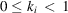. It corresponds to the unit cell used in crystallography for the description of crystal structures in direct space.

Let k be some vector according to (1.5.3.12) and W be the matrices of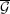. The following definitions are useful:

Definition. The set of all vectorsfulfilling the condition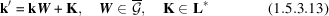is called the orbit of k.

Definition. The set of all matrices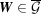for whichforms a group which is called the little co-group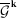of k. The vector k is called general if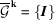; otherwise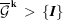and k is called special.

In words: The k vector is called general if its little co-group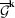is the identity of. Otherwise,is a non-trivial subgroup of the point groupof,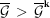, and k is called a special vector of the reciprocal space.

Equation (1.5.3.14)for k resembles the equationby which the fixed points of the symmetry operationof a symmorphic space groupare determined. Indeed, the orbits of k defined by (1.5.3.13) correspond to the point orbits of, the little co-groupof k corresponds to the site-symmetry group of that point X whose coordinateshave the same values as the vector coefficientsof k.

Consider the coset decomposition ofrelative to.

Definition. Ifis a set of coset representatives ofrelative to, then the setis called the star of k and the vectorsare called the arms of the star.

The number of arms of the star of k is equal to the order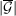of the point groupdivided by the order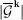of the symmetry groupof k. If k is general, then there arevectors from the orbit of k in each fundamental region andarms of the star. If k is special with little co-group, then the number of arms of the star of k and the number of k vectors in the fundamental region from the orbit of k is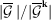.

Definition. The group of all elementsfor which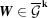is called the little groupof k.

The analogue of the little groupis rarely considered in crystallography.

All symmetry operations ofmay be obtained as combinations of an operation that leaves the origin fixed with a translation of L, i.e. are of the kind. We now define the analogous group for the k vectors. Whereasis a realization of the corresponding abstract group in direct (point) space, the group to be defined will be a realization of it in reciprocal (vector) space.

Definition. The group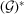which is the semidirect product of the point groupand the translation group of the reciprocal latticeofis called the reciprocal-space group of.

By this definition, the reciprocal-space groupis isomorphic to a symmorphic space group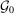. The elements ofare the operations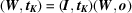withand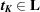with the coefficients of K. In order to emphasize thatis a group acting on reciprocal space and not the inverse of a space group (whatever that may mean) we insert a hyphen -' between reciprocal' and `space'.

From the definition ofit follows that space groups of the same type define the same type of reciprocal-space group. Moreover, asdoes not depend on the column parts of the space-group operations, all space groups of the same arithmetic crystal class determine the same type of; for arithmetic crystal class see Section 1.5.3.2. Following Wintgen (1941), the types of reciprocal-space groupsare listed for the arithmetic crystal classes of space groups, i.e. for all space groups, in Appendix A1.5.1.

### References

Wintgen, G. (1941). Zur Darstellungstheorie der Raumgruppen. Math. Ann. 118, 195–215.